java实现开根号的运算java基础 同时被 2 个专栏收录102 篇文章 2 订阅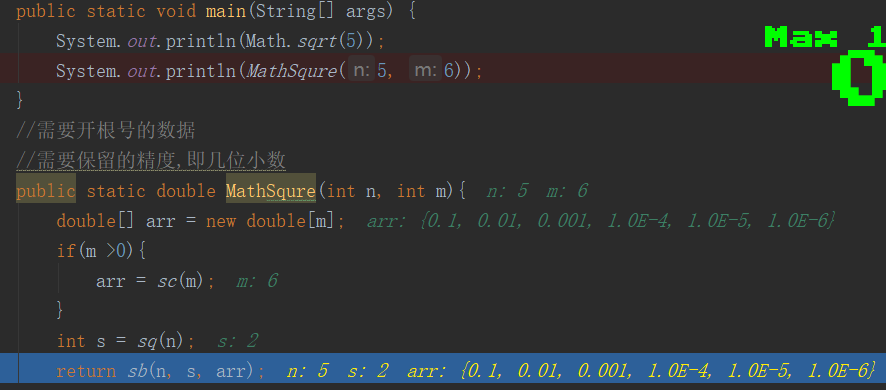import java.math.BigDecimal;

/**
* @Auther: liuhaidong
* Data: 2020/4/21 0021、20:55
* Description:
* @version: 1.0
*/
public class Test6 {
public static void main(String[] args) {
System.out.println(Math.sqrt(5));
System.out.println(MathSqure(5, 6));
}
//需要开根号的数据
//需要保留的精度,即几位小数
public static double MathSqure(int n, int m){
double[] arr = new double[m];
if(m >0){
arr = sc(m);
}
int s = sq(n);
return sb(n, s, arr);
}
/**
* 计算要保留几位小数
* @param m
* @return
*/
public static double[] sc(int m){
double[] arr = new double[m];
int num = 0;
while(num != m){
double f = 1;
for(int i=0;i<=num;i++){
f = f*10;
}
arr[num] = 1/f;
num++;
}
return arr;
}
/**
* 计算整数位
* @param n
* @return
*/
public static int sq(int n){
if( n == 1){
return 1;
}
int tmp = 0;
for(int i=1;i<=n/2+1;i++){
if(i*i == n){
tmp = i;
break;
}
if(i*i > n){
tmp = i-1;
break;
}
}
return tmp;
}

/**
* 开根号
* @param n
* @param j
* @param arr
* @return
*/
public static double sb(int n, double j, double[] arr){
double tmp = j;
for(int p=0;p<arr.length;p++){
if(p>0){
j = tmp;//计算过后的值（整数位+小数位的和，赋值给j，下面继续运算）
}
for(int i=1;i<=9;i++){//小数位只有九位{0.1,0.2,0.3,0.4,0.5,0.6,0.7,0.8,0.9}
tmp = i*arr[p]+j;//i*arr[p],相当于每次加0.1,0.2 ...
if(tmp*tmp == n){
return tmp;
}
if(tmp*tmp >n){
//避免丢失精度
BigDecimal c1 = new BigDecimal(Double.toString(tmp));
BigDecimal c2 = new BigDecimal(Double.toString(arr[p]));
tmp = c1.subtract(c2).doubleValue();
break;
}
}
}
return tmp;
}
}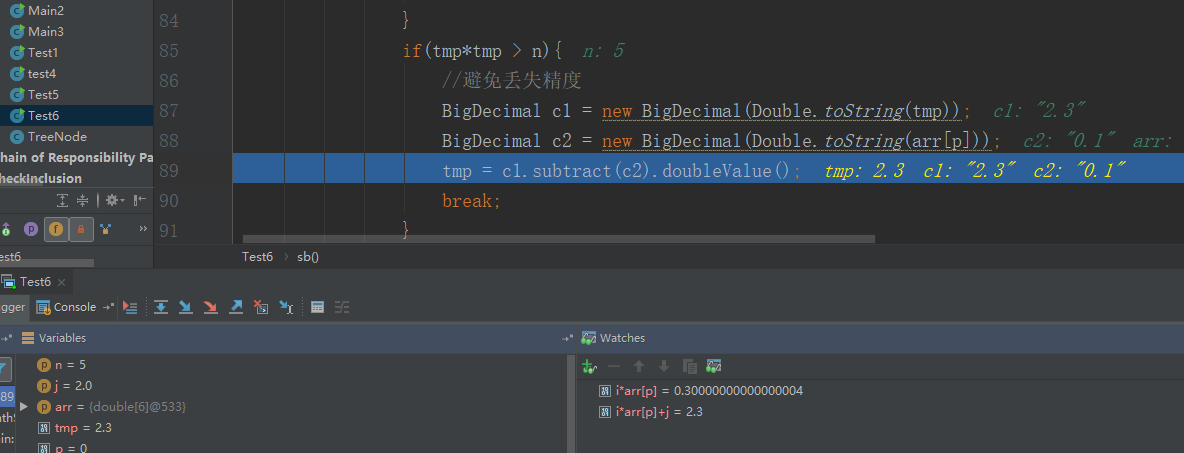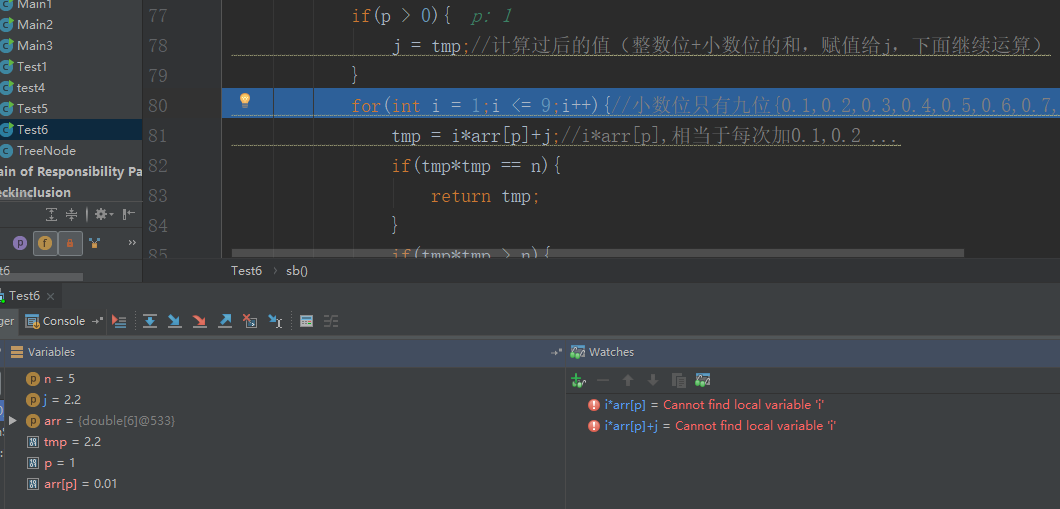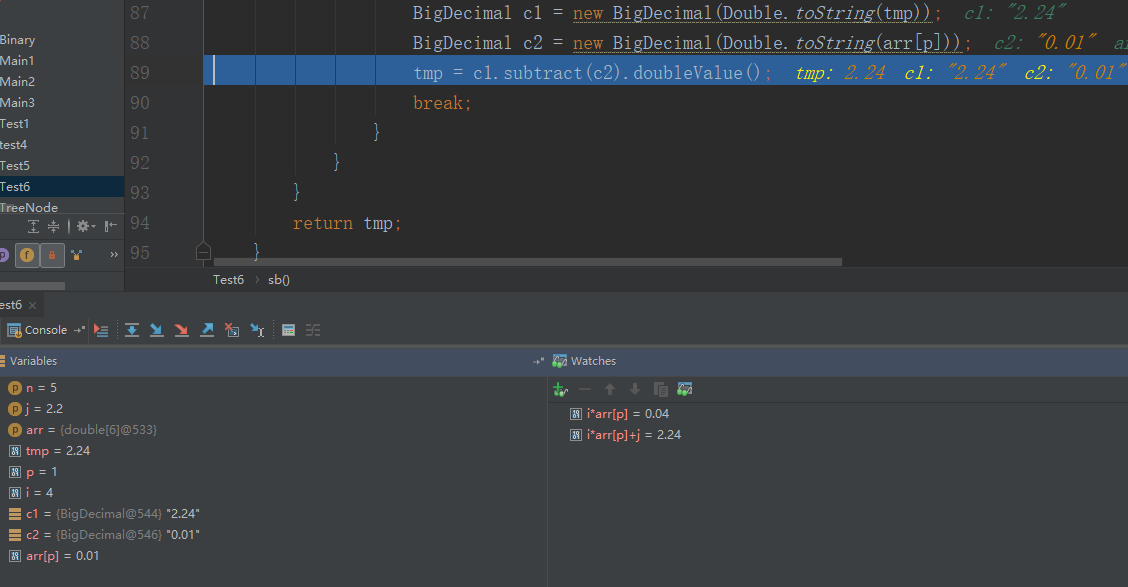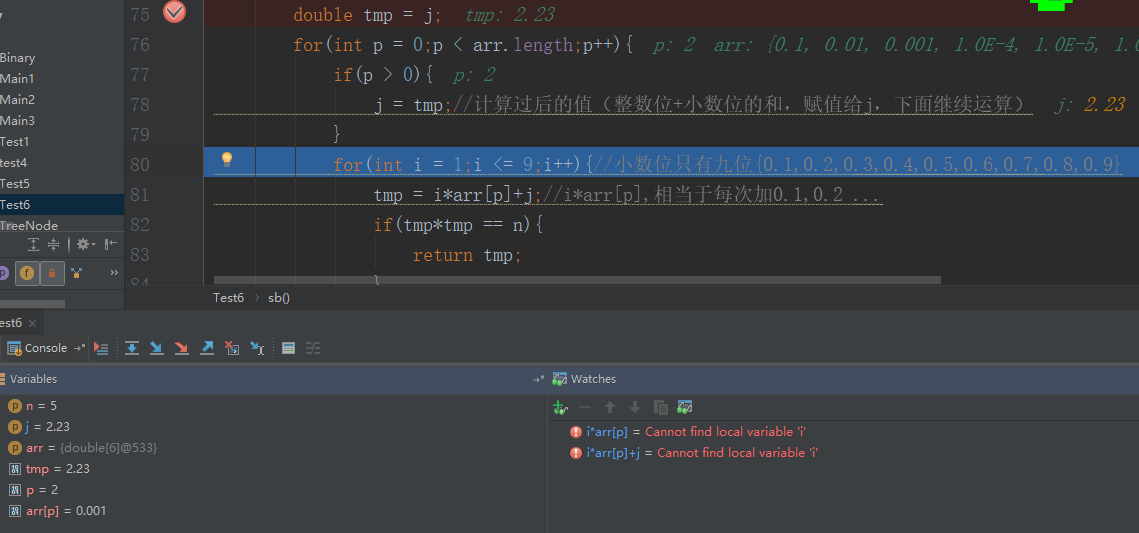依次这样做。11-09108

05-031426
11-20261
01-14378
12-218309
11-10631
11-052340
11-2942
02-131154
02-131101
08-222万+
01-212336
02-12861
09-308415
12-02438
12-131821
10-31109haikuotiankongdong

¥2 ¥4 ¥6 ¥10 ¥20余额支付 (余额：-- )扫码支付获取中扫码支付点击重新获取扫码支付1.余额是钱包充值的虚拟货币，按照1:1的比例进行支付金额的抵扣。
2.余额无法直接购买下载，可以购买VIP、C币套餐、付费专栏及课程。余额充值